## RS Aggarwal Solutions Class 10 Chapter 7 Trigonometric Ratios of Complementary Angles Ex 7

These Solutions are part of RS Aggarwal Solutions Class 10. Here we have given RS Aggarwal Solutions Class 10 Chapter 7 Trigonometric Ratios of Complementary Angles Ex 7.

Other Exercises

Question 1.
Solution:
(i)(ii)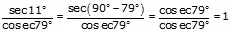(iii)(iv)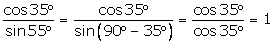(v)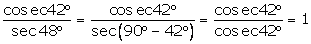(vi)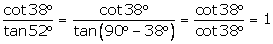Question 2.
Solution:
(i)  LHS = cos81° – sin9°
= cos(90° -9°)- sin9° = sin9° – sin9°
= 0 = RHS

(ii) LHS = tan71° – cot19°
=tan(90° – 19°) – cot19° =cot19° – cot19°
=0 = RHS

(iii) LHS = cosec80° – sec10°
= cosec(90° – 10°) – sec(10°)
= sec10° – sec10° = 0
= RHS

(iv) LHS = cosec72° − tan18°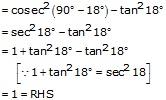(v) LHS = cos75° + cos15°(vi) LHS = tan66° − cot24°(vii) LHS = sin48° + sin42°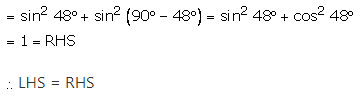(viii) LHS = cos57° − sin33°(ix) LHS = (sin 65° + cos 25°)(sin 65° − cos 25°)Question 3.
Solution:
(i)(ii)(iii)(iv)(v)(vi)Question 4.
Solution:
(i)(ii)(iii)(iv)(v)Question 5.
Solution:
(i)(ii)(iii)(iv)(v)(vi)(vii)Question 6.
Solution:
(i)(ii)(iii)(iv)(v)Question 7.
Solution:
(i)  LHS = sin (70° + θ) − cos (20° − θ)(ii)  LHS = tan (55° − θ) − cot (35° + θ)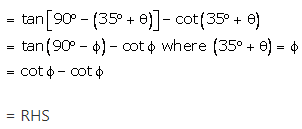(iii)  LHS = cosec (67° + θ) − sec (23° − θ)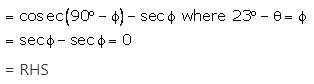(iv)  LHS = cosec (65 °+ θ)  sec  (25° −  θ) − tan (55° − θ) + cot (35° + θ)(v)  LHS = sin (50° + θ ) − cos (40° − θ) + tan 1° tan 10° tan 80° tan 89°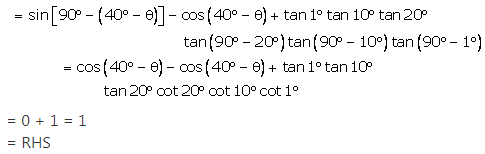Question 8.
Solution:
(i)(ii)(iii)(iv)Question 9.
Solution:Question 10.
Solution:Question 11.
Solution:Question 12.
Solution:Question 13.
Solution:Question 14.
Solution:Question 15.
Solution:We hope the RS Aggarwal Solutions Class 10 Chapter 7  Trigonometric Ratios of Complementary Angles Ex 7 help you. If you have any query regarding RS Aggarwal Solutions Class 10 Chapter 7  Trigonometric Ratios of Complementary Angles Ex 7, drop a comment below and we will get back to you at the earliest.